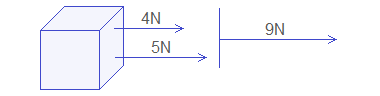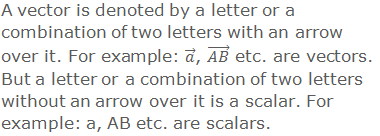# Vectors and it's Components## Vectors: Introduction

The quantities which can be measured are called physical quantities. Some physical quantities have magnitudes only but some physical quantities have magnitudes as well as directions.

********************

10 Math Problems officially announces the release of Quick Math Solver, an Android App on the Google Play Store for students around the world.

********************

Let us study the addition of some physical quantities. For example, if we have two rectangles of areas 6cm2 and 8cm2, the total areas of these two rectangles is (6 + 8)cm2 i.e. 14cm2.If two forces 4N and 5N act on a body, what is the total force acting on the body, 9N or 1N? Its answer is uncertain unless their directions are known. If two forces have same direction the total force on the body is 4N + 5N = 9N.If two forces have opposite direction the total force on the body is 5N – 4N = 1N.In the calculation of some physical quantities, the direction has very important role.

## Scalars and Vectors

Scalar Quantity: A physical quantity which can be measured with its magnitude only is known as a scalar quantity. For example: mass, length, area, volume etc.

Vector Quantity: A physical quantity which can be measured with its magnitude as well as direction is known as a vector quantity. For example: velocity, acceleration, force etc.

## Vector Notation## Representation of a vector

A vector is generally represented by a directed line segment. The length of the line segment is chosen according to some scale to represent the magnitude and the arrow to represent the direction of the vector.## Directed Line Segments

“If two points of a line are taken, one as the initial point and another as the terminal point, then it is called a directed line segment.”If the length and direction of two directed line segments are same, they are called equivalent directed line segments or equal directed line segments. In the figure given below## Position Vector

A point P(x, y) determines its position with reference to origin O(0, 0). Join OP, draw PNOX, so ON = x and PN = y.## Vectors in Terms of Components

Let P(x1, y1) and Q(x2, y2) be two points on the plane. From P and Q draw PMOX and QNOX and again draw PRQN.### Worked Out Examples

Example 1: Find the vectors represented by the following line segments in component form.Solution: In the above graph,Example 2: Represent the following vectors by the directed line segments.#### Do you have any questions regarding the vectors and it's components?

You can ask your questions or problems here, in the comment section below.